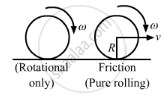Advertisement Remove all ads

# A Uniform Wheel of Radius R is Set into Rotation About Its Axis at an Angular Speed ω. this Rotating Wheel is Now Placed on a Rough Horizontal Surface with Its Axis Horizontal. - Physics

Sum

A uniform wheel of radius R is set into rotation about its axis at an angular speed ω. This rotating wheel is now placed on a rough horizontal surface with its axis horizontal. Because of friction at the contact, the wheel accelerates forward and its rotation decelerates till the wheel starts pure rolling on the surface. Find the linear speed of the wheel after it starts pure rolling.

Advertisement Remove all ads

#### SolutionInitial angular momentum,

$L = I\omega$

$= \frac{1}{2}m R^2 \omega$

Angular momentum after it starts pure rolling,

$L' = I\omega' + m\left( v \times R \right)$

$= \frac{1}{2}m R^2 \left( \frac{v}{R} \right) + mvR$

$= \frac{3}{2}mVR$

As no external torque is applied, angular momentum will be conserved.

Therefore, we have

L = L'

$\Rightarrow \frac{1}{2}m R^2 \omega = \frac{3}{2}mvR$

$\Rightarrow v = \frac{\omega R}{3}$

Is there an error in this question or solution?
Advertisement Remove all ads

#### APPEARS IN

HC Verma Class 11, 12 Concepts of Physics 1
Chapter 10 Rotational Mechanics
Q 81 | Page 200
Advertisement Remove all ads

#### Video TutorialsVIEW ALL 

Advertisement Remove all ads
Share
Notifications

View all notifications

Forgot password?